Courses

# Test: Problems On Ages - 2

## 20 Questions MCQ Test General Aptitude for GATE 2020 | Test: Problems On Ages - 2

Description
This mock test of Test: Problems On Ages - 2 for Banking Exams helps you for every Banking Exams entrance exam. This contains 20 Multiple Choice Questions for Banking Exams Test: Problems On Ages - 2 (mcq) to study with solutions a complete question bank. The solved questions answers in this Test: Problems On Ages - 2 quiz give you a good mix of easy questions and tough questions. Banking Exams students definitely take this Test: Problems On Ages - 2 exercise for a better result in the exam. You can find other Test: Problems On Ages - 2 extra questions, long questions & short questions for Banking Exams on EduRev as well by searching above.
QUESTION: 1

### The sum of the present ages of a son and his father is 60 years. Six years ago, father's age was five times the age of the son. After 6 years, what will be son's age?

Solution:

Let the present age of the son = x Then, present age of the father = ( 60 − x ) Six years ago father's age was 5 times the age of the son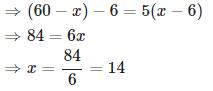Son's age after 6 years = x + 6 = 14 + 6 = 20

QUESTION: 2

### Kiran is younger than Bineesh by 7 years and their ages are in the respective ratio of 7 : 9. How old is Kiran?

Solution:

Let age of Kiran and Bineesh be 7x and  9x  respectively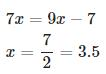Kiran's age = 7 x = 7 × 3.5 = 24.5

QUESTION: 3

### Six years ago, the ratio of the ages of Vimal and Saroj was 6 : 5 . Four years hence, the ratio of their ages will be 11 : 10 . What is Saroj's age at present?

Solution:

Given that, six years ago, the ratio of the ages of Vimal and Saroj = 6 : 5
Hence we can assume that age of Vimal six years ago = 6x
age of Saroj six years ago = 5x
After 4 years, the ratio of their ages = 11 : 10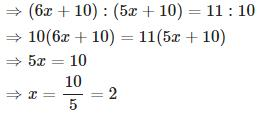Saroj's present age

= ( 5x + 6 ) = 5 x 2 + 6 = 16

QUESTION: 4

At present, the ratio between the ages of Shekhar and Shobha is 4 : 3 . After 6 years, Shekhar's age will be 26 years. Find out the age of Shobha at present?

Solution:

After 6 years, Shekhar's age will be 26 years
Therefore, Present age of Shekhar = 26 − 6 = 20
Let present age of Shobha = x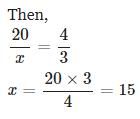QUESTION: 5

My brother is 3 years elder to me. My father was 28 years of age when my sister was born while my mother was 26 years of age when I was born. If my sister was 4 years of age when my brother was born, then what was the age of my father when my brother was born?

Solution:

Let my age = x
Then
My brother's age = x + 3
My mother's age = x + 26
My sister's age = ( x + 3 ) + 4 = x + 7 My father's age = ( x + 7 ) + 28 = x + 35
Age of my father when my brother was born = x + 35 − ( x + 3 ) = 32

QUESTION: 6

The present ages of A,B and C are in proportions 4 : 7 : 9 . Eight years ago, the sum of their ages was 56 . What are their present ages (in years)?

Solution:

Let present age of A,B and C be 4 x , 7 x and 9 x respectively.
(4x−8)+(7x−8)+(9x−8)=56
⇒20x=80
⇒x=4
Hence present age of A, B and C are
4 × 4 , 7 × 4 and 9 × 4 respectively.
i.e., 16 , 28 and 36 respectively.

QUESTION: 7

A person's present age is two-fifth of the age of his mother. After 8 years, he will be one-half of the age of his mother. What is the present age of the mother?

Solution:

Let present age of the person =x
Then, present age of the mother = 5x/2
Given that, after  8  years, the person will be one-half of the age of his mother.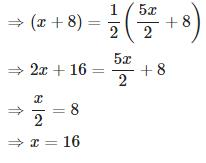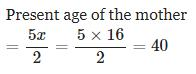QUESTION: 8

A is as much younger than B and he is older than C. If the sum of the ages of B and C is 50 years, what is definitely the difference between B and A's age?

Solution:

Age of C < Age of A < Age of B

Given that sum of the ages of B and C is 50 years.

Now we need to find out (B's age - A's age). But this cannot be determined with the given data.

QUESTION: 9

Sobha's father was 38 years of age when she was born while her mother was 36 years old when her brother four years younger to her was born. What is the difference between the ages of her parents?

Solution:

Let Sobha's age = x and
her brother's age = x − 4
Sobha's father's age = x + 38
Sobha's mother's age = ( x − 4 ) + 36 = x + 32
Sobha's father's age - Sobha's mother's age
=(x+38)−(x+32)=6

QUESTION: 10

The age of father 10 years ago was thrice the age of his son. Ten years hence, father's age will be twice that of his son. What is the ratio of their present ages?

Solution:

Let age of the son before 10 years = x and  age of the father before 10 years = 3 x
(3x + 20 ) = 2 ( x + 20 )
⇒ x = 20
Age of the son at present = x + 10 = 20 + 10 = 30
Age of the father at present = 3 x + 10 = 3 × 20 + 10 = 70
Required ratio = 70 : 30 = 7 : 3

QUESTION: 11

The ages of two persons differ by 16 years. 6 years ago, the elder one was 3 times as old as the younger one. What are their present ages of the elder person?

Solution:

Let present age of the elder person = x and
present age of the younger person = x − 16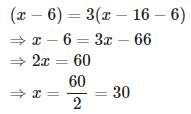QUESTION: 12

Present age of a father is 3 years more than three times the age of his son. Three years hence, father's age will be 10 years more than twice the age of the son. What is father's present age?

Solution:

Let the present age the son = x

Then, present age of the father = 3 x + 3

Given that, three years hence, father's age will be 10 years more than twice the age of the son

⇒ ( 3 x + 3 + 3 ) = 2 ( x + 3 ) + 10

⇒ x = 10

Father's present age

= 3 x + 3 = 3 × 10 + 3 = 33

QUESTION: 13

Kamal was 4 times as old as his son 8 years ago. After 8 years, Kamal will be twice as old as his son. Find out the present age of Kamal.

Solution:

Let age of the son before 8 years = x

Then, age of Kamal before 8 years ago = 4x

After 8 years, Kamal will be twice as old as his son

⇒ 4 x + 16 = 2 ( x + 16 )

⇒ x = 8

Present age of Kamal = 4 x + 8 = 4 × 8 + 8 = 40

QUESTION: 14

If 6 years are subtracted from the present age of Ajay and the remainder is divided by 18 , then the present age of Rahul is obtained. If Rahul is 2 years younger to Denis whose age is 5 years, then what is Ajay's present age?

Solution:

Present age of Denis = 5 years

Present age of Rahul = 5 − 2 = 3

Let present age of Ajay = x

Then, present age of Rahul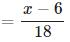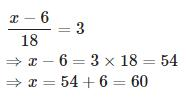QUESTION: 15

The ratio of the age of a man and his wife is 4 : 3 . At the time of marriage the ratio was 5 : 3 and After 4 years this ratio will become 9 : 7 . How many years ago were they married?

Solution:

Let the present age of the man and his wife be 4 x and 3 x respectively.

After 4 years this ratio will become 9 : 7 ⇒ ( 4 x + 4 ) : ( 3 x + 4 ) = 9 : 7
⇒ 7 ( 4 x + 4 ) = 9 ( 3 x + 4 )
⇒ 28 x + 28 = 27 x + 36
⇒ x = 8

Present age of the man = 4 x = 4 × 8 = 32
Present age of his wife = 3 x = 3 × 8 = 24
Assume that they got married before t years. Then,
( 32 − t ) : ( 24 − t ) = 5 : 3
⇒ 3 ( 32 − t ) = 5 ( 24 − t )
⇒ 96 − 3 t = 120 − 5 t
⇒ 2 t = 24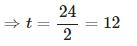QUESTION: 16

The product of the ages of Syam and Sunil is 240 . If twice the age of Sunil is more than Syam's age by 4 years, what is Sunil's age?

Solution:

Let age of Sunil = x
and age of Syam = y
xy = 240 ⋯ ( 1 )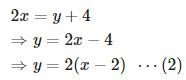Substituting equation ( 2 ) in equation ( 1 ) . We get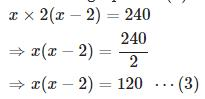We got a quadratic equation to solve.
Always time is precious and objective tests measure not only how accurate you are but also how fast you are. We can solve this quadratic equation in the traditional way. But it is more easy to substitute the values given in the choices in the quadratic equation (equation 3 ) and see which choice satisfy the equation.
Here, option A is 10 . If we substitute that value in the quadratic equation, x ( x − 2 ) = 10 × 8 which is not equal to 120
Now try option B which is 12 . If we substitute that value in the quadratic equation, x ( x − 2 ) = 12 × 10 = 120 . See, we got that x = 12
Hence Sunil's age = 12
(Or else, we can solve the quadratic equation by factorization as,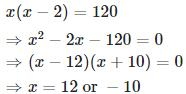Since x is age and cannot be negative, x = 12
Or by using quadratic formula as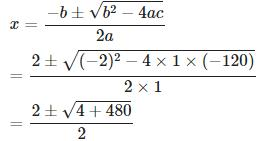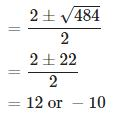Since age is positive, x = 12

QUESTION: 17

One year ago, the ratio of Sooraj's and Vimal's age was 6 : 7 respectively. Four years hence, this ratio would become 7 : 8 . How old is Vimal?

Solution:

Let the age of Sooraj and Vimal, 1 year ago, be 6 x and 7 x respectively.
Given that, four years hence, this ratio would become 7 : 8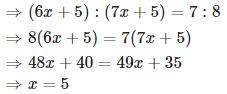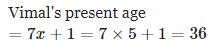QUESTION: 18

The total age of A and B is 12 years more than the total age of B and C. C is how many year younger than A?

Solution:

Given that, A + B = 12 + B + C

⇒ A - C = 12

Therefore, C is younger than A by 12 years

QUESTION: 19

Sachin's age after 15 years will be 5 times his age 5 years back. Find out the present age of Sachin?

Solution:

Let present age of Sachin = x . Then,
( x + 15 ) = 5 ( x − 5 )
⇒ 4 x = 40
⇒ x = 10

QUESTION: 20

Sandeep's age after six years will be three-seventh of his father's age. Ten years ago the ratio of their ages was 1 : 5 . What is Sandeep's father's age at present?

Solution:

Let the age of Sandeep and his father before 10 years be x and 5 x respectively.
Given that Sandeep's age after six years will be three-seventh of his father's age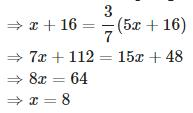Sandeep's father's present age
= 5 x + 10 = 5 × 8 + 10 = 50